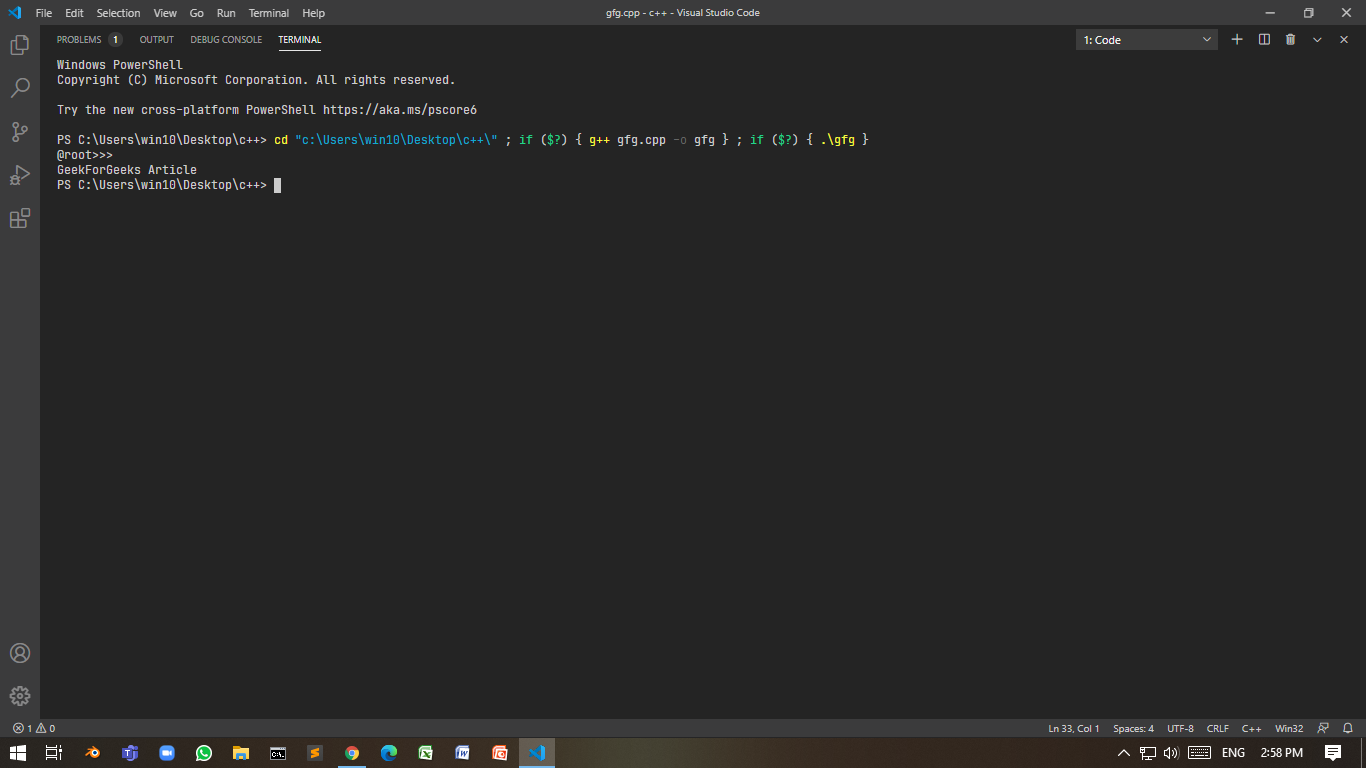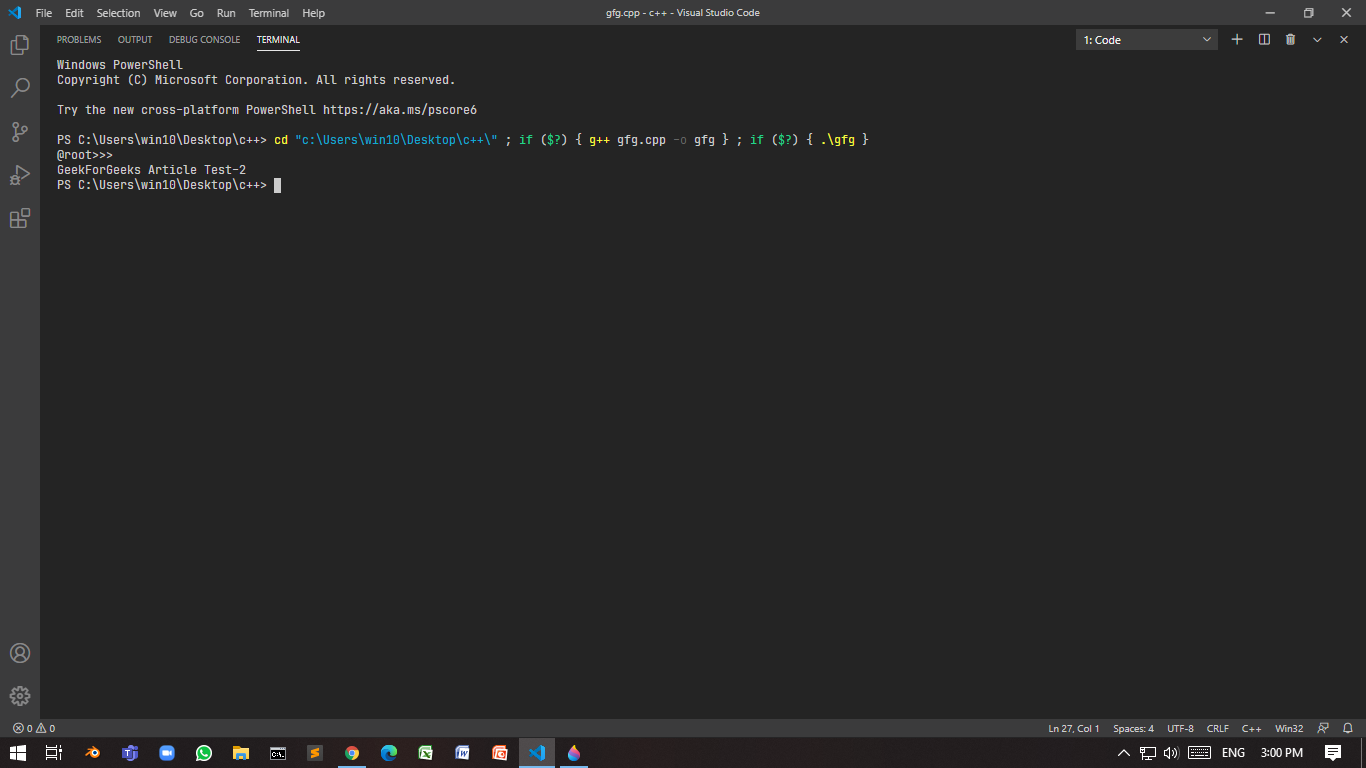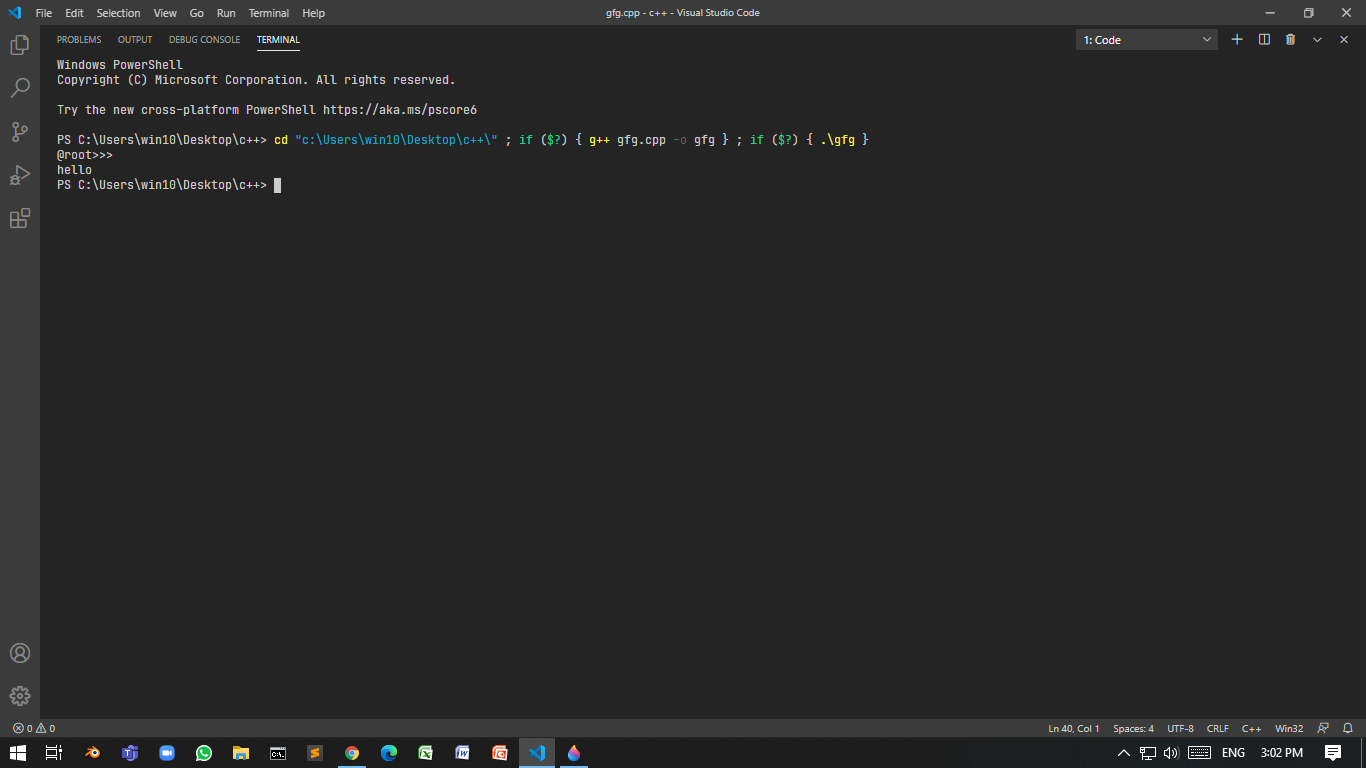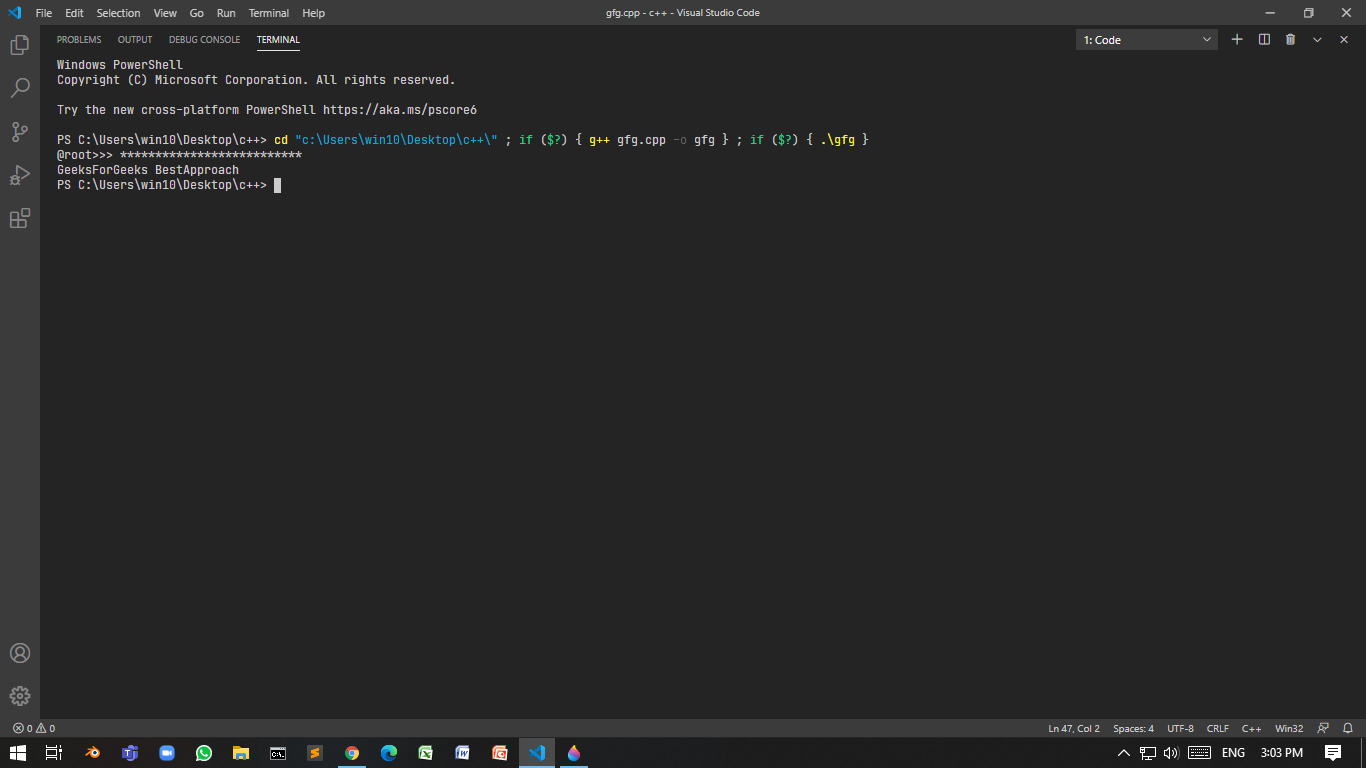Open in App
Not now

# Taking password as input in C++

• Difficulty Level : Hard
• Last Updated : 22 Nov, 2022

There are two methods in which input can be taken in a more secure way:

• Do not display any content.
• Display a special character such as an asterisk instead of actual content.

In this method, input content will be invisible. This can be implemented in two ways:

### using <windows.h>:

Program 1:

Below is the program where console mode is set to enable, echo input, and reset the console mode:

## C++

 `// C++ program to take the input ` `// invisibly ` `#include ` `#include ` `using` `namespace` `std; ` ` `  `// Function take password and ` `// reset to console mode ` `std::string takePasswdFromUser() ` `{ ` `    ``HANDLE` `hStdInput ` `        ``= GetStdHandle(STD_INPUT_HANDLE); ` `    ``DWORD` `mode = 0; ` ` `  `    ``// Create a restore point Mode ` `    ``// is know 503 ` `    ``GetConsoleMode(hStdInput, &mode); ` ` `  `    ``// Enable echo input ` `    ``// set to 499 ` `    ``SetConsoleMode( ` `        ``hStdInput, ` `        ``mode & (~ENABLE_ECHO_INPUT)); ` ` `  `    ``// Take input ` `    ``string ipt; ` `    ``getline(cin, ipt); ` ` `  `    ``// Otherwise next cout will print ` `    ``// into the same line ` `    ``cout << endl; ` ` `  `    ``// Restore the mode ` `    ``SetConsoleMode(hStdInput, mode); ` ` `  `    ``return` `ipt; ` `} ` ` `  `// Driver Code ` `int` `main() ` `{ ` `    ``string input; ` `    ``cout << ``"@root>>> "``; ` ` `  `    ``// Function Call ` `    ``input = takePasswdFromUser(); ` ` `  `    ``// Print the input ` `    ``cout << input << endl; ` `}`

Output:### using <conio.h>:

For this getch() is used. This function takes a character input from user without buffer and doesn’t wait for the user to press “return” key.

Program 2:

Below is the C++ program to demonstrate the use of getch() in conio.h:

## C++

 `// C++ program to demonstrate the ` `// use of getch() ` `#include ` `#include ` `using` `namespace` `std; ` ` `  `// Function using getch() ` `std::string takePasswdFromUser() ` `{ ` `    ``string ipt = ``""``; ` `    ``char` `ipt_ch; ` `    ``while` `(``true``) { ` `        ``ipt_ch = getch(); ` ` `  `        ``// Check whether user enters ` `        ``// a special non-printable ` `        ``// character ` `        ``if` `(ipt_ch < 32) { ` `            ``cout << endl; ` `            ``return` `ipt; ` `        ``} ` `        ``ipt.push_back(ipt_ch); ` `    ``} ` `} ` ` `  `// Driver Code ` `int` `main() ` `{ ` `    ``string input; ` `    ``cout << ``"@root>>> "``; ` ` `  `    ``// Function call ` `    ``input = takePasswdFromUser(); ` `    ``cout << input << endl; ` `}`

Output:Drawback: The user can’t clear the response made earlier. When backspace is pressed, the input is returned.

Program 3:

Below is the C++ program to demonstrate the solution to the above drawback:

## C++

 `// C++ program to demonstrate the ` `// solution of above drawback ` `#include ` `#include ` `using` `namespace` `std; ` ` `  `// Enumerator ` `enum` `TT_Input { ` ` `  `    ``// ASCII code of backspace is 8 ` `    ``BACKSPACE = 8, ` `    ``RETURN = 32 ` `}; ` ` `  `// Function accepting password ` `std::string takePasswdFromUser() ` `{ ` `    ``string ipt = ``""``; ` `    ``char` `ipt_ch; ` `    ``while` `(``true``) { ` `        ``ipt_ch = getch(); ` ` `  `        ``if` `(ipt_ch < TT_Input::RETURN ` `            ``&& ipt_ch != TT_Input::BACKSPACE) { ` `            ``cout << endl; ` `            ``return` `ipt; ` `        ``} ` ` `  `        ``// Check whether the user ` `        ``// pressed backspace ` `        ``if` `(ipt_ch == TT_Input::BACKSPACE) { ` ` `  `            ``// Check if ipt is empty or not ` `            ``if` `(ipt.length() == 0) ` `                ``continue``; ` `            ``else` `{ ` ` `  `                ``// Removes last character ` `                ``ipt.pop_back(); ` ` `  `                ``continue``; ` `            ``} ` `        ``} ` `        ``ipt.push_back(ipt_ch); ` `    ``} ` `} ` ` `  `// Driver Code ` `int` `main() ` `{ ` `    ``string input; ` `    ``cout << ``"@root>>> "``; ` ` `  `    ``// Function call ` `    ``input = takePasswdFromUser(); ` `    ``cout << input << endl; ` `}`### Hiding the password by a special character(*):

The idea is to use the library <conio.h> here to hide password with asterisk(*). Below is the C++ program using conio.h to hide the password using *:

Program 4:

## C++

 `// C++ program to hide the password ` `// using *(asterik) ` `#include ` `#include ` `using` `namespace` `std; ` ` `  `// Enumerator ` `enum` `IN { ` ` `  `    ``// 13 is ASCII for carriage ` `    ``// return ` `    ``IN_BACK = 8, ` `    ``IN_RET = 13 ` ` `  `}; ` ` `  `// Function that accepts the password ` `std::string takePasswdFromUser( ` `    ``char` `sp = ``'*'``) ` `{ ` `    ``// Stores the password ` `    ``string passwd = ``""``; ` `    ``char` `ch_ipt; ` ` `  `    ``// Until condition is true ` `    ``while` `(``true``) { ` ` `  `        ``ch_ipt = getch(); ` ` `  `        ``// if the ch_ipt ` `        ``if` `(ch_ipt == IN::IN_RET) { ` `            ``cout << endl; ` `            ``return` `passwd; ` `        ``} ` `        ``else` `if` `(ch_ipt == IN::IN_BACK ` `                 ``&& passwd.length() != 0) { ` `            ``passwd.pop_back(); ` ` `  `            ``// Cout statement is very ` `            ``// important as it will erase ` `            ``// previously printed character ` `            ``cout << ``"\b \b"``; ` ` `  `            ``continue``; ` `        ``} ` ` `  `        ``// Without using this, program ` `        ``// will crash as \b can't be ` `        ``// print in beginning of line ` `        ``else` `if` `(ch_ipt == IN::IN_BACK ` `                 ``&& passwd.length() == 0) { ` `            ``continue``; ` `        ``} ` ` `  `        ``passwd.push_back(ch_ipt); ` `        ``cout << sp; ` `    ``} ` `} ` ` `  `// Driver Code ` `int` `main() ` `{ ` `    ``string input; ` `    ``cout << ``"@root>>> "``; ` ` `  `    ``// Function call ` `    ``input = takePasswdFromUser(); ` `    ``cout << input << endl; ` `}`My Personal Notes arrow_drop_up
Related Articles